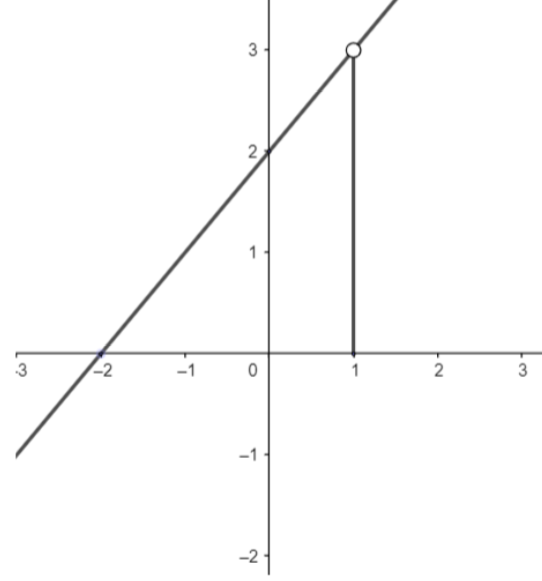Courses
Courses for Kids
Free study material
Free LIVE classes
MoreLIVE
Join Vedantu’s FREE Mastercalss

# As a final illustration, find the value of $\underset{x\to 1}{\mathop{\lim }}\,f\left( x \right)$ where$f\left( x \right)=\left\{ \begin{matrix} x+2,x\ne 1 \\ 0,x=1 \\\end{matrix} \right.$Verified
363.3k+ views
Hint: The limit of a function exists only if left hand limit and right hand limit exist and both are equal.

Also, the value of the limit will be equal to the value of the right hand limit and (or) the left hand limit.
We know, the limit of a function exists only if the left hand limit and right hand limit exist and both are equal.
So, first, we will find the left hand limit of the function $f(x)$ at $x=1$.
We know, the left hand limit of a function$f\left( x \right)$ at the point $x=a$ is given as
$\underset{h\to 0}{\mathop{\lim }}\,f\left( a-h \right)$
So , the left hand limit of the function$f\left( x \right)$ at the point$x=1$ is given as
$\underset{h\to 0}{\mathop{\lim }}\,f\left( 1-h \right)$
Now, we know h is a very small quantity and subtracting h from 1 will give a number less than 1 but very close to 1. But, since the number is not equal to 1, so, $f\left( 1-h \right)=1-h+2$
So, $\underset{h\to 0}{\mathop{\lim }}\,f\left( 1-h \right)=\underset{h\to 0}{\mathop{\lim }}\,1-h+2=1-0+2=3$
Now, we will find the right hand limit of the function$f\left( x \right)$ at the point $x=1$.
We know right hand limit of a function $f\left( x \right)$ at $x=a$ is given by
$R.H.L=\underset{h\to 0}{\mathop{\lim }}\, f\left( a+h \right)$
So, the right hand limit of the function $f\left( x \right)$ at the point $x=1$ is given by
$\underset{h\to 0}{\mathop{\lim }}\, f\left( 1+h \right)$
Now, we know h is a very small number. So, 1+h will be slightly greater than 1 and not equal to 1. So, $f\left( 1+h \right)=1+h+2$
So, $\underset{h\to 0}{\mathop{\lim }}\,f\left( 1+h \right)=\underset{h\to 0}{\mathop{\lim }}\,\left( 1+h+2 \right)=3$
We can clearly see that both the left hand limit and the right hand limit of the function $f\left( x \right)$ exist at the point $x=1$.
Also, the left hand limit and the right hand limit of the function $f\left( x \right)$ at the point $x=1$ are equal.
Since, the value of $L.H.L=R.H.L$ at $x=1$, hence, limit of the function $f\left( x \right)$ exists at $x=1$ and the value of limit of the function $f\left( x \right)$at $x=1$ is $3$.
So, $\underset{x\to 1}{\mathop{\lim }}\,f\left( x \right)=3$

Note: Graph of $f\left( x \right)=\left\{ \begin{matrix} x+2,x\ne 1 \\ 0,x=1 \\ \end{matrix} \right.$ is given asFrom the graph we can see, just slightly to the left of $x=1$ and slightly to the right of $x=1$, the value of $f\left( x \right)$ is approximately equal to 3.
Hence, the value of $\underset{x\to 1}{\mathop{\lim }}\,f\left( x \right)=3$.
Last updated date: 25th Sep 2023
Total views: 363.3k
Views today: 8.63k